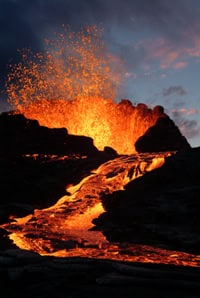Volcanoes have been a source of both fascination and fear for millennia. In the year AD 79, the eruption of Mt Vesuvius laid waste to the Roman town of Pompeii, burying its citizens alive under ash and lava. Today, scientists monitor volcanoes like Vesuvius for signs of impending outbursts, hoping to avoid a modern-day disaster. Using data from instruments in the field, these volcanologists can create mathematical models to predict when the next eruption is due, allowing people in the area to evacuate before it’s too late.

### Poisson predictionOne of the simplest volcanic models is the Poisson process, which uses records of historical eruptions to predict what will happen in the future. This model has many applications in science and business as it describes the time between random events that occur at an average rate. For example, a supermarket might know they receive an average of 100 customers per hour, but those customers arrive at random times throughout the hour. In the case of volcanoes, we don’t know how many eruptions there are per year, but volcanologists can use historical data to produce an estimate.

The Poisson process model says that the number of eruptions per year follows a Poisson distribution with parameter. This means we can use the formula for the Poission distribution to calculate the probability of a certain number of eruptions in a year:Here X represents the number of eruptions pear year, and x is the particular number of eruptions we are interested in calculating a probability for. The model also tells us that the time between eruptions follows an exponential distribution, with the probability of an eruption occurring at time T within the next t years being:But what is? In the supermarket example we could keep a record of how many customers visit during a whole week to work out the average per hour and thus set= 100, but most volcanoes have only erupted a dozen or so times throughout human history. With so little data to inform their decision, how can volcanologists choose a good value for? The answer is a mathematical trick called maximum likelihood estimation, or MLE.

### Is that likely?Maximum likelihood estimation lets statisticians ask questions in reverse. A simple probability example might ask you to calculate the odds of flipping a coin 10 times and getting 8 heads, given there is an equal chance of heads or tails. Using MLE, we can ask which probability between 0 and 1 is most likely to result in 8 heads out of 10. If the answer is way off 0.5, we might start to suspect something is wrong with the coin!

The general idea of MLE is to construct a likelihood function based on a) sample data from whatever you’re modelling and b) the parameter of the model you’re using. You can then use calculus or numerical methods to find which value of the parameter maximizes the function. For a full explanation of the maths, you can read this introduction to MLE (from Kings College London, but it’s enough to just keep in mind the basic concept: given the data we have, which value of the parameter in our model is most likely to produce those results?

Volcanologists can use this principle by calculating the value ofmost likely to produce a known pattern of eruption times. It involves a lot of number crunching, but the end result is a model for predicting the next eruption. Suppose the data shows that= 0.004 is the most likely value, meaning eruptions occur at a rate of 0.004 per year. On average you would expect to see one eruption every 250 years, but what’s the probability of an eruption in the next 5 years? Our model says:which means P(T < 5) = 0.02, or 2%. Not too worrying then, but what about the next 100 years? P(T < 100) = 0.33, or 33%, which is a bit more concerning. And it’s almost certain there will be an eruption in the next 1000 years, because P(T < 1000) = 0.98, or 98%.

The Poisson process is a fairly simple model that assumes the rate of eruptions is constant for a particular volcano, but volcanologists use many other statistical techniques in their work. Time-series analysis helps search for trends or clustering in the frequency of eruptions, identifying when a volcano is becoming more active, while fractal analysis looks at how often eruptions of different strengths occur. Studying volcanology combines strong mathematical and statistical skills with fieldwork in exotic locations around the world, and most important of all, it can actually save lives.